#### 期刊菜单

Several Problems in Michelson Interferometer and Its Interference

Abstract: The Michelson interferometer is an important precision optical research instrument that uses the split-amplitude method to obtain coherent double beams to achieve interference. It has been in-vented since 1881 for nearly 140 years. However, in practical applications, there are still some differences in the understanding of the role of individual components, and there are some controversies about the concept of some interference in the light involved. Based on the principle and concept of the most basic light interference, this paper combines Young’s interference, equidistant interference, equal-thickness interference and Michelson’s interference for comparative analysis based on relevant literature data, and gives a reasonable viewpoint in order to calm down the existing disagreements and disputes.

1. 引言

2019年7月24日至27日，由教育部高等学校大学物理课程教学指导委员会、教育部高等学校物理学类专业教学指导委员会、中国物理学会物理教学委员会主办的“2019年全国高等学校物理基础课程教育学术研讨会”和“2019年全国高等学校物理基础课程青年教师讲课比赛决赛”在云南师范大学呈贡校区隆重举行。根据教育部高等学校大学物理课程教学指导委员会和教育部高等学校物理学类专业教学指导委员会的活动规划和安排，今年的青年教师讲课比赛的课型为实验课教学的角逐。

1) 迈克尔逊干涉是属于杨氏干涉还是属于等倾干涉？

2) 迈克尔逊干涉仪中的补偿板是否是必须的？

3) 迈克尔逊干涉的实验光路图应该是单线光路还是复线光路？

2. 光的干涉原理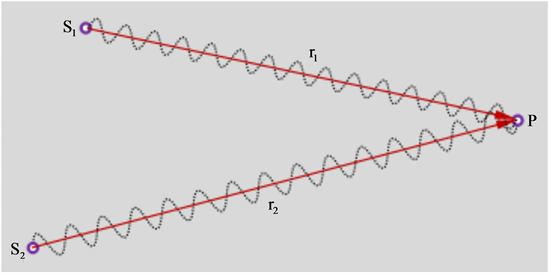Figure 1. The principle of interference of light

${y}_{10}={A}_{1}\mathrm{cos}\left(\omega t+{\phi }_{1}\right)$ (1)

${y}_{10}={A}_{2}\mathrm{cos}\left(\omega t+{\phi }_{2}\right)$ (2)

${y}_{1}={A}_{1}\mathrm{cos}\left[\omega t+\left({\phi }_{1}-\frac{2\pi {r}_{1}}{\lambda }\right)\right]$ (3)

${y}_{2}={A}_{2}\mathrm{cos}\left[\omega t+\left({\phi }_{2}-\frac{2\pi {r}_{2}}{\lambda }\right)\right]$ (4)

${y}_{1}$${y}_{2}$ 的合成的振动方程为：

$y={y}_{1}+{y}_{2}=A\mathrm{cos}\left(\omega t+\phi \right)$ (5)

$A=\sqrt{{A}_{1}^{2}+{A}_{2}^{2}+2{A}_{1}{A}_{2}\mathrm{cos}\left({\phi }_{2}-{\phi }_{1}-2\pi \frac{{r}_{2}-{r}_{1}}{\lambda }\right)}$ (6)

$\phi =\mathrm{arctan}\frac{{A}_{1}\mathrm{sin}\left({\phi }_{1}-\frac{2\pi {r}_{1}}{\lambda }\right)+{A}_{2}\mathrm{sin}\left({\phi }_{2}-\frac{2\pi {r}_{2}}{\lambda }\right)}{{A}_{1}\mathrm{cos}\left({\phi }_{1}-\frac{2\pi {r}_{1}}{\lambda }\right)+{A}_{2}\mathrm{cos}\left({\phi }_{2}-\frac{2\pi {r}_{2}}{\lambda }\right)}$ (7)

$\Delta \phi =\left[\omega t+\left({\phi }_{2}-\frac{2\pi {r}_{2}}{\lambda }\right)\right]-\left[\omega t+\left({\phi }_{1}-\frac{2\pi {r}_{1}}{\lambda }\right)\right]={\phi }_{2}-{\phi }_{1}-2\pi \frac{{r}_{2}-{r}_{1}}{\lambda }$ (8)

$\Delta \phi =2\pi \frac{{r}_{2}-{r}_{1}}{\lambda }=±2k\pi$ ，即 $\delta ={r}_{2}-{r}_{1}=±k\lambda \text{\hspace{0.17em}}\text{ }\left(k=0,1,2,3,\cdots \right)$ (9)

${A}_{\mathrm{max}}={A}_{1}+{A}_{2}$ (10)

$\Delta \phi =2\pi \frac{{r}_{2}-{r}_{1}}{\lambda }=±\left(2k+1\right)\pi$ ，即 $\delta ={r}_{2}-{r}_{1}=±\left(2k+1\right)\frac{\lambda }{2}\text{\hspace{0.17em}}\text{ }\text{ }\left(k=0,1,2,3,\cdots \right)$ (11)

${A}_{\mathrm{min}}=|{A}_{1}-{A}_{2}|$ (12)

3. 两个典型的光的干涉实例

3.1. 杨氏干涉

1801年，英国物理学家托马斯·杨(1773~1829)在实验室里首次成功地观察到了光的干涉。如图2所示是托马斯·杨的实验  光路，他采用烛光作为光源，烛焰、单缝、双缝和光屏等关于轴线SO对称。其中，单缝的作用是获得线光束，双缝的作用是获得振动情况完全相同的相干光束，图中双缝S1与S2之间的距离d以及 $\theta$ 角在实际实验装置或实验中都是很小的。Figure 2. Optical path of Young’s interference experiment

$\delta =\left({r}_{02}+{r}_{2}\right)-\left({r}_{01}+{r}_{1}\right)={r}_{2}-{r}_{1}\approx d\mathrm{sin}\theta \approx d\mathrm{tan}\theta =d\frac{x}{D}$ (13)

$\delta =d\frac{x}{D}=\left\{\begin{array}{l}±k\lambda ,\text{\hspace{0.17em}}\text{\hspace{0.17em}}\left(合振动极大\right)\\ ±\left(2k+1\right)\frac{\lambda }{2},\text{\hspace{0.17em}}\text{\hspace{0.17em}}\left(合振动极小\right)\end{array}\text{\hspace{0.17em}}\text{\hspace{0.17em}}\left(k=0,1,2,3,\cdots \right)$ (14)

$x=\left\{\begin{array}{l}±k\frac{D\lambda }{d},\text{\hspace{0.17em}}\text{\hspace{0.17em}}\left(明条纹\right)\\ ±\left(2k+1\right)\frac{D\lambda }{2d},\text{\hspace{0.17em}}\text{\hspace{0.17em}}\left(暗条纹\right)\end{array}\text{\hspace{0.17em}}\text{\hspace{0.17em}}\left(k=0,1,2,3,\cdots \right)$ (15)

$\Delta x={x}_{k+1}-{x}_{k}=\frac{D\lambda }{d}$ (16)

3.2. 薄膜干涉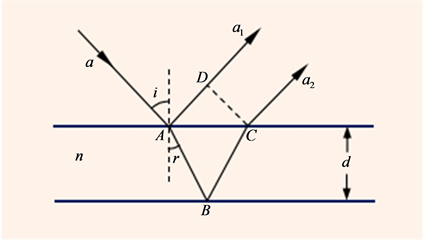Figure 3. Thin-film interference optical path

$\delta =n\left(AB+BC\right)-AD+\frac{\lambda }{2}$ (17)

$\left\{\begin{array}{l}\mathrm{sin}i=n\mathrm{sin}r\\ AD=AC\mathrm{sin}i\\ AB=BC=\frac{d}{\mathrm{cos}r}\\ AC=2d\mathrm{tan}r\end{array}$ (18)

$\delta =2dn\mathrm{cos}r+\frac{\lambda }{2}或\delta =2d\sqrt{{n}^{2}-{\mathrm{sin}}^{2}i}+\frac{\lambda }{2}$ (19)

$\delta =2d\sqrt{{n}^{2}-{\mathrm{sin}}^{2}i}+\frac{\lambda }{2}=\left\{\begin{array}{l}k\lambda ,\text{\hspace{0.17em}}\text{\hspace{0.17em}}\left(k=1,2,3,\cdots \right)\text{\hspace{0.17em}}\text{\hspace{0.17em}}明条纹\\ \left(2k+1\right)\frac{\lambda }{2},\text{\hspace{0.17em}}\text{\hspace{0.17em}}\left(k=0,1,2,3,\cdots \right)\text{\hspace{0.17em}}\text{\hspace{0.17em}}暗条纹\end{array}$ (20)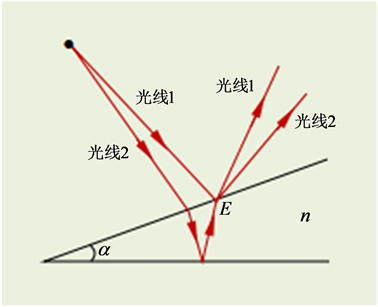Figure 4. Equal-thickness interference of thin-film surface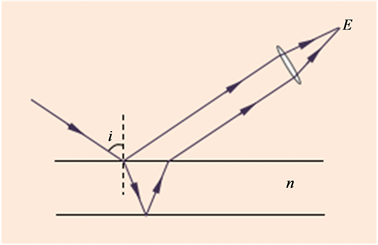Figure 5. Equiclinal interference at infinity

4. 迈克尔逊干涉仪和迈克尔逊干涉

4.1. 迈克尔逊干涉仪结构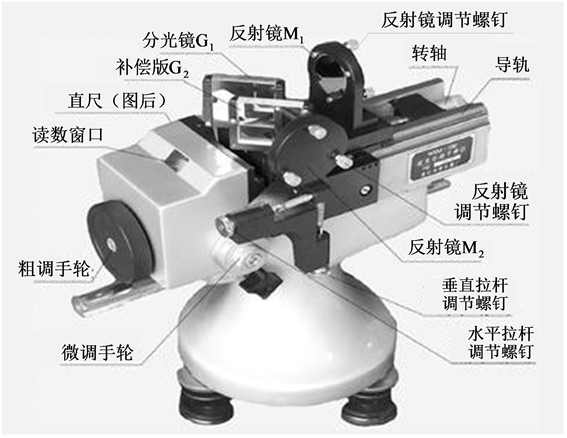Figure 6. Michelson interferometer

4.2. 迈克耳逊干涉原理

$\delta =2d\sqrt{{1}^{2}-{\mathrm{sin}}^{2}i}$ (21)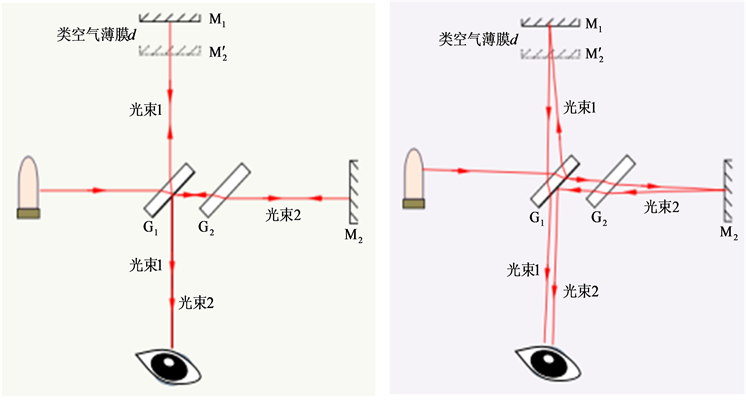(a) 入射光束与分光镜严格成45˚角 (b) 入射光束与分光镜不严格成45˚角

Figure 7. Michelson interference optical path

$\delta =2d\sqrt{{1}^{2}-{\mathrm{sin}}^{2}i}=\left\{\begin{array}{l}k\lambda ,\text{\hspace{0.17em}}\text{\hspace{0.17em}}\left(k=1,2,3,\cdots \right)\text{\hspace{0.17em}}\text{\hspace{0.17em}}明条纹\\ \left(2k+1\right)\frac{\lambda }{2},\text{\hspace{0.17em}}\text{\hspace{0.17em}}\left(k=0,1,2,3,\cdots \right)\text{\hspace{0.17em}}\text{\hspace{0.17em}}暗条纹\end{array}$ (22)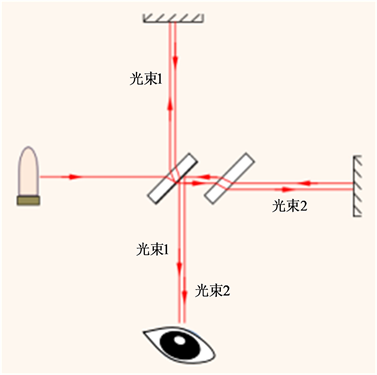Figure 8. Michelson interference conventional error optical path diagram

1) 当M1垂直M2或M1平行 ${{M}^{\prime }}_{\text{2}}$ 时，产生等倾干涉，条纹间距随着M1${{M}^{\prime }}_{\text{2}}$ 之间的距离减小而增大。

2) 当M1不严格垂直M2或M1${{M}^{\prime }}_{\text{2}}$ 之间存在微小夹角时，产生等厚干涉，条纹形状跟M1${{M}^{\prime }}_{\text{2}}$ 之间的夹角决定。

5. 结论

1) 迈克尔逊干涉与薄膜干涉都是采用分振幅法获得相干光束，而杨氏干涉是采用分波阵面法获得相干光束。但迈克尔逊干涉与杨氏干涉或薄膜干涉在概念上没有相互兼容性，因此，关于迈克尔逊干涉是否属于杨氏干涉或薄膜干涉的提法是极不妥当的。

2) 迈克尔逊干涉仪具有既能产生等倾干涉也能产生等厚干涉的特点，因此，不能简单地将迈克尔逊干涉定论为等倾干涉或等厚干涉。

3) 迈克尔逊干涉仪实现干涉过程中，补偿板的作用是避免两相干光束之间出现较大的光程差。但在实践实验中，通过调节干涉臂长度也可以实现两相干光束的不同光程，因此，没有补偿板同样也可以达到所需要求。

4) 迈克尔逊干涉光路并不唯一，至于是单线光路[比如图7(a)]还是复线光路[比如图7(b)]，要根据入射光束跟分束镜等元件之间的位置精密关系的实际情况才能确定，但要避免图8类似的光路。

  陈植. 信息仪中光束提升器改变光矢量振动方向问题研究[J]. 天津纺织工学院学报, 1987, 20(1): 58-61.  王高亮, 孟明, 王强. 基于Mathematica的杨氏双缝干涉实验仿真[J]. 周口师范学院学报, 2018, 35(5): 42-44.  杨庆鑫, 吕天全, 孙敬姝. 托马斯•杨的双缝演示应用于电子干涉实验[J]. 物理通报, 2003(11): 41-43.  陈康. “最美丽”的十大物理实验给我们的启示[J]. 物理通报, 2003(12): 43.  魏茂金, 曹晓琴. 任意入射角情况额外程差的研究[J]. 湖南工学院学报(自然科学版), 2012, 25(4): 37-42.  范希智, 祝霁洺, 易迎彦. 分振幅双光束等倾干涉中半波损失的讨论[J]. 光学仪器, 2012, 34(5): 54-60.  苏瑞洲, 陈小凡, 汤冬华. 光程的概念与薄膜干涉中光程差的表示方法[J]. 高师理科学刊, 2014, 34(34): 43-45.  孙艳, 孙锋, 杨玉孝, 谭玉山. 一种新的膜厚测试技术[J]. 计算技术, 2002(3): 6-9.  赵丽娟, 凌洁华. 薄膜干涉滤光片单色性的研究与应用[J]. 光学仪器, 2007, 29(4): 71-74.  时凯, 苏俊宏, 齐媛. 基于激光外差干涉技术的薄膜厚度测量方法[J]. 应用光学, 2019, 40(3): 473-477.  崔海瑛. 薄膜干涉研究[J]. 大庆师范学院学报, 2018, 38(6): 33-35.  杨广武, 刘枝明, 朱飞, 徐建, 张守超. 便携投影式牛顿环干涉演示仪的研制与应用[J]. 物理与工程, 2018, 28(Z1): 76-80.  秦先明. 大学物理实验[M]. 北京: 高等教育出版社, 2016(1): 184-188.  赵凯华. 新概念物理教程光学[M]. 北京: 高等教育出版社, 2004(1): 130-143.